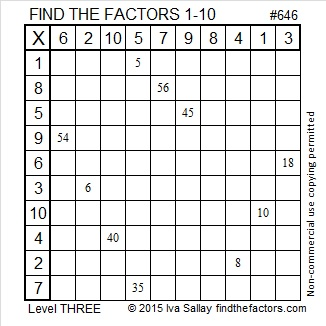1035 is the 23rd Hexagonal Number

1035 is the 23rd hexagonal number because of the way that it can be factored:
2(23²) – 23 = 1035,
(2(23) – 1)23 = 1035
or simply 45(23) = 1035.Starting at the bottom of the hexagonal we see 1 yellow square, 5 green squares, 9 blue squares, 13 purple squares, 17 red squares, and 21 orange squares.

1, 5, 9, 13, 17, 21, . . . is an arithmetic progression or arithmetic sequence. The common difference between the numbers is 4.

The nth hexagonal number is the sum of the first n numbers in that arithmetic progression.
The first few hexagonal numbers form an arithmetic series: 1, 6, 15, 28, 45, 66 and so forth.
1035 is the sum of the first 23 numbers in the progression so it is the 23rd term in the series and the 23rd hexagonal number.

All hexagonal numbers are also triangular numbers. 1035 is the 45th triangular number because 45(46)/2 = 1035.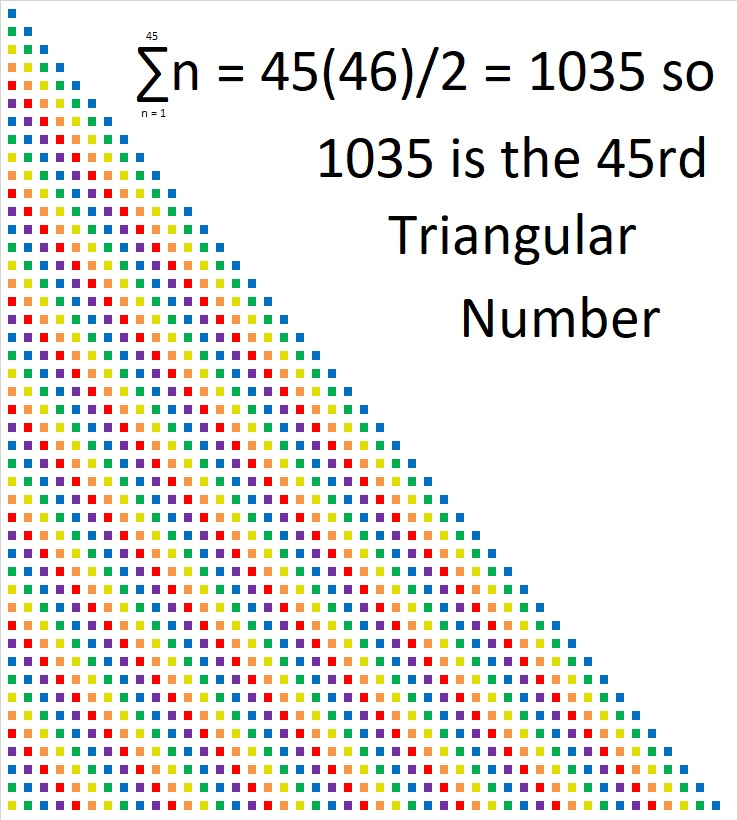Starting in the lower left-hand corner of that triangle we see 1 yellow square, 2 green squares, 3 blue squares, 4 purple squares, 5 red squares, and 6 orange squares.

1, 2, 3, 4, 5, 6, . . .  is the simplest arithmetic progression there is. The common difference is 1.

The nth triangular number is the sum of the first n numbers in that arithmetic progression.

The first few triangular numbers form an arithmetic series: 1, 3,  6, 10, 15, 21, 28, 36, 45, 55, 66 and so forth.  (The blue triangular numbers are also hexagonal numbers.)
1035 is the sum of the first 45 numbers in the progression so it is the 45th term in the series and the 45th triangular number.

1035 is also the hypotenuse of one Pythagorean triple:
621-828-1035 which is (3-4-5) times 207

It is also a leg in several Pythagorean triples including
1035-1380-1725 which is (3-4-5) times 345

• 1035 is a composite number.
• Prime factorization: 1035 = 3 × 3 × 5 × 23, which can be written 1035 = 3² × 5 × 23
• The exponents in the prime factorization are 2, 1, and 1. Adding one to each and multiplying we get (2 + 1)(1 + 1)(1 + 1) = 3 × 2 × 2 = 23. Therefore 1035 has exactly 12 factors.
• Factors of 1035: 1, 3, 5, 9, 15, 23, 45, 69, 115, 207, 345, 1035
• Factor pairs: 1035 = 1 × 1035, 3 × 345, 5 × 207, 9 × 115, 15 × 69, or 23 × 45,
• Taking the factor pair with the largest square number factor, we get √1035 = (√9)(√115) = 3√115 ≈ 32.1714857 Why do Primitive Pythagorean Triples Do That?

29² + 4² = 857, so 857 is the hypotenuse of a Pythagorean triple that just happens to be primitive:

• 232-825-857 which was calculated from 2(29)(4), 29² – 4², 29² + 4².

Now, one of the legs of a primitive Pythagorean triple will be divisible by 4. In this case that number is 232. As I indicated when I wrote 821 Why Do Primitive Pythagorean Triples Do That? The sum of the number divisible by 4 in a primitive Pythagorean triple and the hypotenuse always equals a perfect square. So 232 + 857 = 1089 = 33².

This week I noticed something about the OTHER leg that might be even more amazing! The other leg in the triple, 825, is not divisible by 4, but get this: One half of the sum of a primitive’s hypotenuse and that leg that is NOT divisible by 4 also equals a square number: For example, (857 + 825)/2 = 1681/2 = 841 = 29².

But not only that, one half of the DIFFERENCE of a primitive’s hypotenuse and that leg that is NOT divisible by 4 equals a perfect square, too: (857 – 825)/2 = 32/2 = 16 = 4².

Now 29² and 4² look a little familiar to me today. Hmm… We’ve seen those two numbers together earlier in this post!

It seems that for Primitive Pythagorean Triples, what goes around comes around!

Check out the sums and difference of these other primitive Pythagorean triples: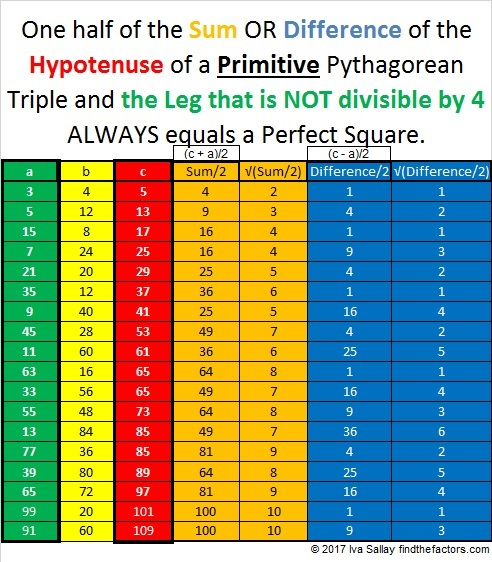Go ahead, try it with any other primitive triple on this list or otherwise!

If we let s = √(Sum/2) and let d = √(Difference/2), then we can rewrite some Pythagorean triple like this:

• s² – d², 2sd, s² + d², so s² and d² are REALLY significant perfect squares!

Besides being the hypotenuse of a primitive Pythagorean triple, here are some other facts about the number 857:

857 is the sum of three consecutive prime numbers: 281 + 283 + 293.

857 is also the sum of the nineteen prime numbers from 11 to 83.

• 857 is a prime number.
• Prime factorization: 857 is prime.
• The exponent of prime number 857 is 1. Adding 1 to that exponent we get (1 + 1) = 2. Therefore 857 has exactly 2 factors.
• Factors of 857: 1, 857
• Factor pairs: 857 = 1 × 857
• 857 has no square factors that allow its square root to be simplified. √857 ≈ 29.27456

How do we know that 857 is a prime number? If 857 were not a prime number, then it would be divisible by at least one prime number less than or equal to √857 ≈ 29.3. Since 857 cannot be divided evenly by 2, 3, 5, 7, 11, 13, 17, 19, 23, or 29, we know that 857 is a prime number.

Here’s another way we know that 857 is a prime number: Since its last two digits divided by 4 leave a remainder of 1, and 29² + 4² = 857 with 29 and 4 having no common prime factors, 857 will be prime unless it is divisible by a prime number Pythagorean triple hypotenuse less than or equal to √857 ≈ 29.3. Since 853 is not divisible by 5, 13, 17, or 29, we know that 857 is a prime number.

821 Why Do Primitive Pythagorean Triples Do That?

The most famous Pythagorean triple is 3-4-5.

Perhaps you know that of the three numbers in EVERY Pythagorean triple at least one of them will be divisible by 3, at least one of them will be divisible by 4, and at least one of them will be divisible by 5. That’s obvious for triple 3-4-5.

Here’s another example: Pythagorean triple 11-60-61. Two of those numbers are prime numbers, yet 60 is divisible by 3, 4, AND 5.

If the Pythagorean triple is a primitive, something else amazing happens:That’s amazing all by itself, but that’s only part of the picture. Let’s look at the complete picture specifically using the triple with hypotenuse 821:

• 25² + 14² = 821
• 429² + 700² = 821²
• 429700821 can be calculated from 25² – 14², 2(25)(14), 25² + 14²
• 821 + 700 = 1521
• √1521 = 39 which is 25 + 14
• 429 ÷ 39 = 11

WHY does this happen to primitive Pythagorean triples?

It happened for 429700821 because 700 82125² + 14² + 2(25)(14) = (25 + 14)².

After all (a + b)² = a² +2ab + b² is always true.

And it can be rearranged: (a + b)² = a² + b² +2ab.

You can use a similar proof whenever the element of the Primitive triple that is divisible by 4 can be expressed as 2ab.

************

But it is a different, and perhaps simpler, story for many triples such as 94041 which was calculated using (9)(1), (9² – 1²)/2, (9² + 1²)/2.

In that case 40 + 41(9² – 1²)/2 + (9² + 1²)/2 = (9² – 1² + 9² + 1²)/2 = 9².

You can use a similar proof whenever the element of the Primitive triple that is divisible by 4 can be expressed as (a² – b²)/2.

************

Go ahead, take ANY primitive Pythagorean triple. Add the leg that is divisible by 4 to its hypotenuse. You will get a perfect square. Here’s a few more examples: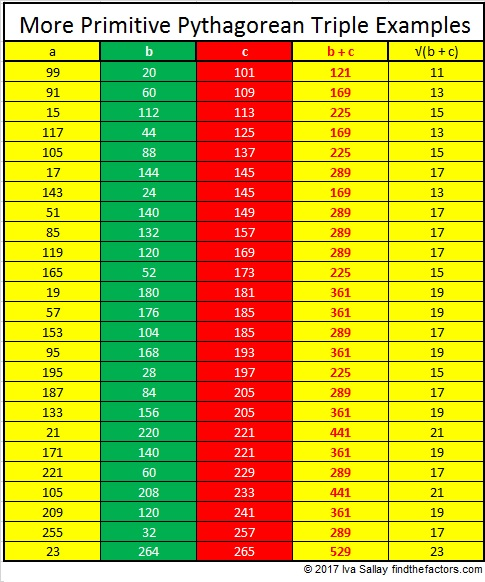Similarly primitive triples that have been multiplied by a square number will also produce a perfect square, but you’ll have to be careful which leg you add to the hypotenuse if you multiplied by a square number that is a multiple of 4.

For example, 9(3-4-5) = 27-36-45 and 36 + 45 = 81, a square number.

But 4(3-4-5) = 12-16-20 so each number is divisible by 4. Note that 16 + 20 = 36, a square number, but 12 + 20 does not.

Here’s a few other essential facts about the number 821:

All of the odd numbers between 820 and 830, except 825, are prime numbers. Thus 821, 823, 827, 829 is the fourth prime decade.

• 821 is a prime number.
• Prime factorization: 821 is prime.
• The exponent of prime number 821 is 1. Adding 1 to that exponent we get (1 + 1) = 2. Therefore 821 has exactly 2 factors.
• Factors of 821: 1, 821
• Factor pairs: 821 = 1 x 821
• 821 has no square factors that allow its square root to be simplified. √821 ≈ 28.65309756.

How do we know that 821 is a prime number? If 821 were not a prime number, then it would be divisible by at least one prime number less than or equal to √821 ≈ 28.7. Since 821 cannot be divided evenly by 2, 3, 5, 7, 11, 13, 17, 19, or 23, we know that 821 is a prime number.Here’s another way we know that 821 is a prime number: Since its last two digits divided by 4 leave a remainder of 1, and 25² + 14² = 821 with 25 and 14 having no common prime factors, 821 will be prime unless it is divisible by a prime number Pythagorean triple hypotenuse less than or equal to √821 ≈ 28.7. Since 821 is not divisible by 5, 13, or 17, we know that 821 is a prime number.

767 is the hypotenuse of a Pythagorean triple, but…

• 767 is a composite number.
• Prime factorization: 767 = 13 x 59
• The exponents in the prime factorization are 1 and 1. Adding one to each and multiplying we get (1 + 1)(1 + 1) = 2 x 2 = 4. Therefore 767 has exactly 4 factors.
• Factors of 767: 1, 13, 59, 767
• Factor pairs: 767 = 1 x 767 or 13 x 59
• 767 has no square factors that allow its square root to be simplified. √767 ≈ 27.69476.Because 13 is one of its factors, 767 is the hypotenuse of Pythagorean triple 295-708-767, but 767 will never be part of the solution to one of these Pythagorean triple puzzles because it is greater than 100. Every side and hypotenuse in these puzzles must be a whole number less than 100. Together the numbers in the puzzle must form Pythagorean triples. Can you solve the puzzle?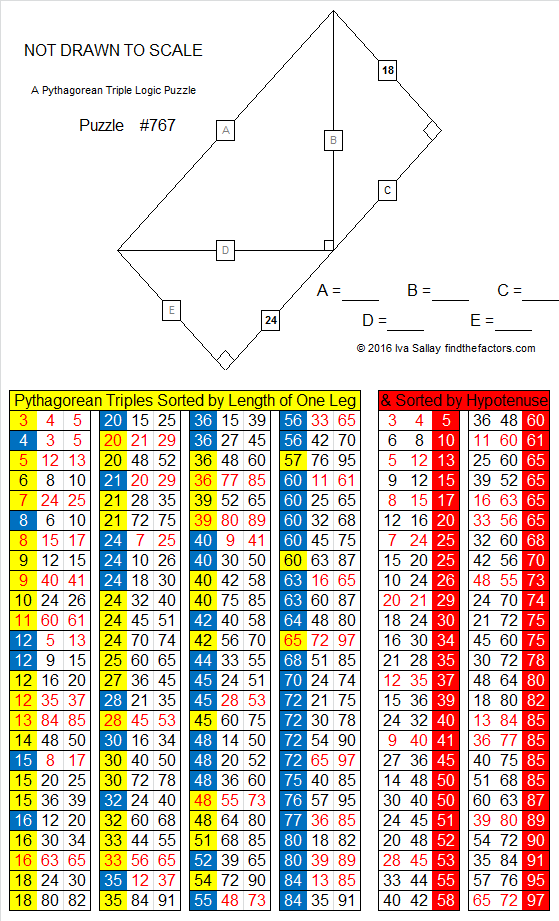Print the puzzles or type the solution on this excel file: 12 Factors 2016-02-25

————————————-

Here’s a little more about the number 767:

767 is a palindrome in two different bases:

• 767 in BASE 10; note that 7(100) + 6(10) + 7(7) = 767.
• 2B2 in BASE 17 (B = 11 base 10); note that 2(289) + 11(17) + 2(1) = 767.

Wikipedia informs us that 767 is also the 9th Thabit number.

————————————-

Simplifiable Square Roots up to √765

• 765 is a composite number.
• Prime factorization: 765 = 3 x 3 x 5 x 17, which can be written 765 = (3^2) x 5 x 17
• The exponents in the prime factorization are 2, 1, and 1. Adding one to each and multiplying we get (2 + 1)(1 + 1)(1 + 1) = 3 x 2 x 2 = 12. Therefore 765 has exactly 12 factors.
• Factors of 765: 1, 3, 5, 9, 15, 17, 45, 51, 85, 153, 255, 765
• Factor pairs: 765 = 1 x 765, 3 x 255, 5 x 153, 9 x 85, 15 x 51, or 17 x 45
• Taking the factor pair with the largest square number factor, we get √765 = (√9)(√85) = 3√85 ≈ 27.658633.765 is the 300th number whose square root can be simplified! Here are three tables with 100 simplifiable square roots each showing all the simplifiable square roots up to √765. When three or more consecutive numbers have simplifiable square roots, I highlighted them.That’s 300 simplifiable square roots found for the first 765 counting numbers. 300 ÷ 765 ≈ 0.392, so 39.2% of the numbers so far have simplifiable square roots.

Today’s puzzle is a whole lot less complicated than all that, so give it a try!Print the puzzles or type the solution on this excel file: 10 Factors 2016-02-04

Logical steps to find the solution are in a table at the bottom of the post.

—————————————

Here are some other fun facts about the number 765:

765 is made from three consecutive numbers so it is divisible by 3. The middle of those numbers is 6 so 765 is also divisible by 9.

765 can be written as the sum of two squares two different ways:

• 27² + 6² = 765
• 21² + 18² = 765

Its other two prime factors, 5 and 17, have a remainder of 1 when divided by 4 so 765² can be written as the sum of two squares FOUR different ways, two of which contain other numbers that use the same digits as 765. Also notice that 9 is a factor of each number in the corresponding Pythagorean triples.

• 117² + 756² = 765²
• 324² + 693² = 765²
• 360² + 675² = 765²
• 459² + 612² = 765²

765 can also be written as the sum of three squares four different ways:

• 26² + 8² + 5² = 765
• 22² + 16² + 5² = 765
• 20² + 19² + 2² = 765
• 20² + 14² + 13² = 765

765 is a palindrome in two different bases:

• 1011111101 BASE 2; note that 1(512) + 0(256) + 1(128) + 1(64) + 1(32) + 1(16) + 1(8) + 1(4) + 0(2) + 1(1) = 765.
• 636 BASE 11; note that 6(121) + 3(11) + 6(1) = 765.

—————————————What Kind of Shape is 703 in?

• 703 is a composite number.
• Prime factorization: 703 = 19 x 37
• The exponents in the prime factorization are 1 and 1. Adding one to each and multiplying we get (1 + 1)(1 + 1) = 2 x 2 = 4. Therefore 703 has exactly 4 factors.
• Factors of 703: 1, 19, 37, 703
• Factor pairs: 703 = 1 x 703 or 19 x 37
• 703 has no square factors that allow its square root to be simplified. √703 ≈ 26.514147.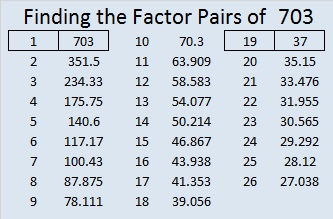703 is a fascinating number:As shown in that graphic, since the square of 703 can be broken apart and added back together to become itself again, 703 is the 7th Kaprekar number. Thank you OEIS.org for that fun fact.

What kind of shape is 703 in?

703 is the 37th triangular number: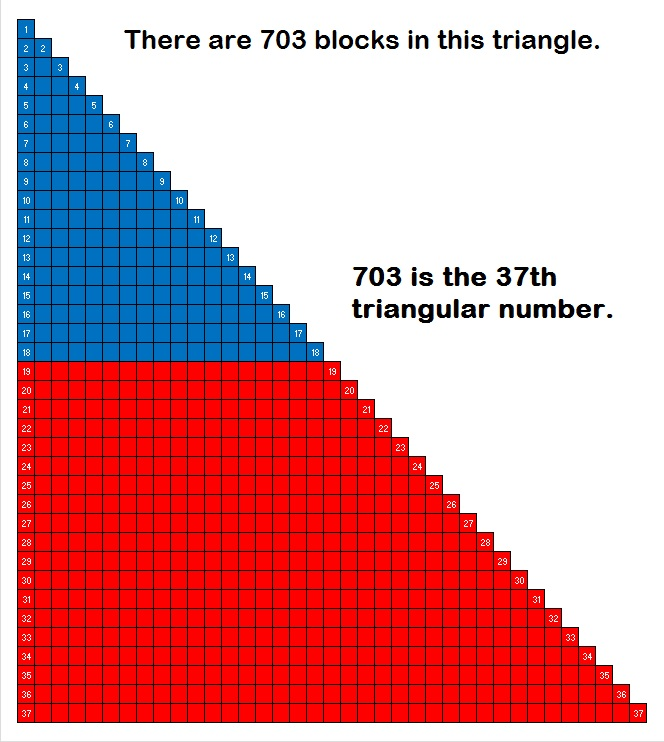703 is a triangular number because 37(38)/2 = 703. If we move part of the triangle, those blocks can be neatly rearranged into this rectangle:About half of all triangular numbers are also hexagonal numbers. 703 is the 19th hexagonal number because (19⋅2)(19⋅2 – 1)/2 = 703.

In the past I found counting the dots in graphics of larger hexagonal numbers to be a nightmare, but yesterday I saw a small graphic for hexagonal number 28 that used different colors for each expansion. I challenged myself to make a graphic showing that 703 is a hexagonal number. I made it in excel using o’s in different colors. When I finished, I told excel to replace the o’s with •’s. Excel informed me that it made 703 replacements.  Then I adjusted the size of the cells to make the hexagon smaller. I also attempted to make the outer-most hexagon look as symmetrical as possible.Hexagonal numbers are not symmetrical the way hexagonal snowflakes are. I thought it would be cool to make 703 into a snowflake, but I didn’t succeed because 703 is just too big and it lacks symmetry. Nevertheless, I must share these directions to make snowflakes that are indeed 6-sided. (Using paper dinner napkins instead of regular paper makes folding and cutting them much simpler.)

703 is a palindrome in three different bases:

• 383 base 14; note that 3(196) + 8(14) + 3(1) = 703
• 111 base 26; note that 1(676) + 1(26) + 1(1) =703
• JJ base 36; note that J is equivalent to 19 in base 10 and 19(36) + 19(1) = 703

Since 37 is one of its factors, 703 is the hypotenuse of the Pythagorean triple we see in this triangle: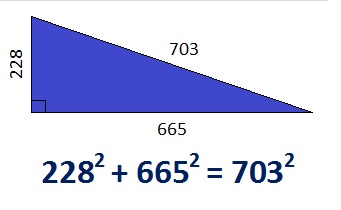If you divide each number in the triple by 19, you’ll get the primitive Pythagorean triple 12-35-37.

703 is also a leg in some Pythagorean triple triangles:

• 504-703-865 (a primitive generated from 2(28)(9), 28² – 9², 28² + 9²) Note that 28 + 9 = 37 and 28 – 9 = 19. Both 37 and 19 are prime factors of 703.
• 703-6660-6697
• 703-12996-13015
• 703-247104-247105 (another primitive)661 Candy Corn

25² + 6² = 661

661 is the hypotenuse of the primitive Pythagorean triple 300-589-661 which was calculated using 2(25)(6), 25² – 6², 25² + 6².

661 is also the sum of the three prime numbers from 211 to 227. What is the prime number in the middle of the sum?

This Find the Factors puzzle is supposed to look like a piece of candy corn. 🙂Print the puzzles or type the solution on this excel file: 10 Factors 2015-10-26

Here’s the puzzle without the possibly distracting color: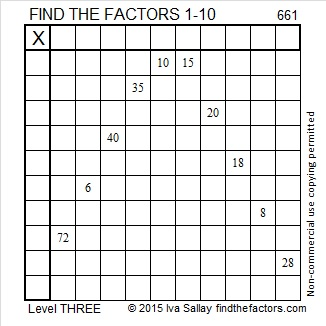————————————

• 661 is a prime number. 659 and 661 are twin primes.
• Prime factorization: 661 is prime.
• The exponent of prime number 661 is 1. Adding 1 to that exponent we get (1 + 1) = 2. Therefore 661 has exactly 2 factors.
• Factors of 661: 1, 661
• Factor pairs: 661 = 1 x 661
• 661 has no square factors that allow its square root to be simplified. √661 ≈ 25.70992.How do we know that 661 is a prime number? If 661 were not a prime number, then it would be divisible by at least one prime number less than or equal to √661 ≈ 25.7. Since 661 cannot be divided evenly by 2, 3, 5, 7, 11, 13, 17, 19, or 23, we know that 661 is a prime number.

Here’s another way we know that 661 is a prime number: Since 25² + 6² = 661, and 25 and 6 have no common prime factors, 661 will be prime unless it is divisible by a primitive Pythagorean hypotenuse less than or equal to √661 ≈ 25.7. Since 661 is not divisible by 5, 13, or 17, we know that 661 is a prime number.

—————————————————————————————————

A Logical Approach to solve a FIND THE FACTORS puzzle: Find the column or row with two clues and find their common factor. (None of the factors are greater than 10.)  Write the corresponding factors in the factor column (1st column) and factor row (top row).  Because this is a level three puzzle, you have now written a factor at the top of the factor column. Continue to work from the top of the factor column to the bottom, finding factors and filling in the factor column and the factor row one cell at a time as you go.655 and Level 6

655  is the hypotenuse of the Pythagorean triple 393-524-655. What is the greatest common factor of those three numbers?

655 is a leg in exactly three triples. One of them is primitive; the rest are not. Which is which?

• 655-1572-1703
• 655-42900-42905
• 655-214512-214513

Which of 655’s factors are the greatest common factors of each of the two that are not primitive?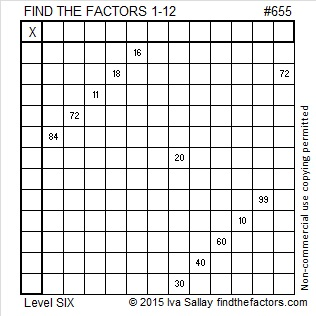Print the puzzles or type the solution on this excel file: 12 Factors 2015-10-19

—————————————————————————————————

• 655 is a composite number.
• Prime factorization: 655 = 5 x 131
• The exponents in the prime factorization are 1 and 1. Adding one to each and multiplying we get (1 + 1)(1 + 1) = 2 x 2 = 4. Therefore 655 has exactly 4 factors.
• Factors of 655: 1, 5, 131, 655
• Factor pairs: 655 = 1 x 655 or 5 x 131
• 655 has no square factors that allow its square root to be simplified. √655 ≈ 25.592968.—————————————————————————————————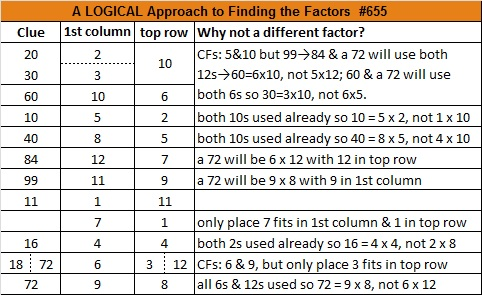650 is the sum of all the clues in this Level 1 puzzle

1² + 2² + 3² + 4² + 5² + 6² +7² + 8² + 9² +10² + 11² + 12²  = 650

Thus 650 is the 12th square pyramidal number and can be calculated using 12(12 +1)(2⋅12 + 1)/6.

If you add up all the clues in today’s Find the Factors puzzle, you will get the number 650. However, if you print the puzzle from the excel file, one of the clues is missing because it isn’t needed to find the solution.

650 is the hypotenuse of seven Pythagorean triples!

• 72-646-650
• 160-630-650
• 182-624-650
• 250-600-650
• 330-560-650
• 390-520-650
• 408-506-650

Can you find the greatest common factor of each triple? Each greatest common factor will be one of the factors of 650 listed below the puzzle.

650 is the hypotenuse of so many Pythagorean triples because it is divisible by 5, 13, 25, 65, and 325 which are also hypotenuses of triples. The smallest three numbers to be the hypotenuses of at least 7 triples are 325, 425, and 650.

Since 25 x 26 = 650, we know that (25-1)(26 + 1) = 650 – 2. Thus 24 x 27 = 648.Print the puzzles or type the solution on this excel file: 12 Factors 2015-10-19

—————————————————————————————————

• 650 is a composite number.
• Prime factorization: 650 = 2 x 5 x 5 x 13, which can be written 650 = 2 x (5^2) x 13
• The exponents in the prime factorization are 1, 2, and 1. Adding one to each and multiplying we get (1 + 1)(2 + 1)(1 + 1) = 2 x 3 x 2 = 12. Therefore 650 has exactly 12 factors.
• Factors of 650: 1, 2, 5, 10, 13, 25, 26, 50, 65, 130, 325, 650
• Factor pairs: 650 = 1 x 650, 2 x 325, 5 x 130, 10 x 65, 13 x 50, or 25 x 26
• Taking the factor pair with the largest square number factor, we get √650 = (√25)(√26) = 5√26 ≈ 25.495098.—————————————————————————————————

646 and Level 3

646 is the hypotenuse of the Pythagorean triple 304-570-646. What 2-digit number is the greatest common factor of those three numbers?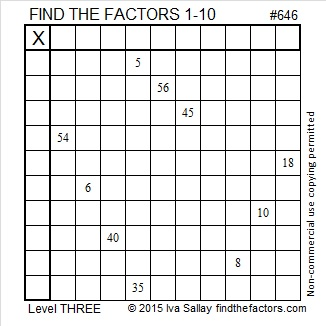Print the puzzles or type the solution on this excel file: 10 Factors 2015-10-12

—————————————————————————————————

• 646 is a composite number.
• Prime factorization: 646 = 2 x 17 x 19
• The exponents in the prime factorization are 1, 1, and 1. Adding one to each and multiplying we get (1 + 1)(1 + 1)(1 + 1) = 2 x 2 x 2 = 8. Therefore 646 has exactly 8 factors.
• Factors of 646: 1, 2, 17, 19, 34, 38, 323, 646
• Factor pairs: 646 = 1 x 646, 2 x 323, 17 x 38, or 19 x 34
• 646 has no square factors that allow its square root to be simplified. √646 ≈ 25.41653.—————————————————————————————————

A Logical Approach to solve a FIND THE FACTORS puzzle: Find the column or row with two clues and find their common factor. (None of the factors are greater than 10.)  Write the corresponding factors in the factor column (1st column) and factor row (top row).  Because this is a level three puzzle, you have now written a factor at the top of the factor column. Continue to work from the top of the factor column to the bottom, finding factors and filling in the factor column and the factor row one cell at a time as you go.